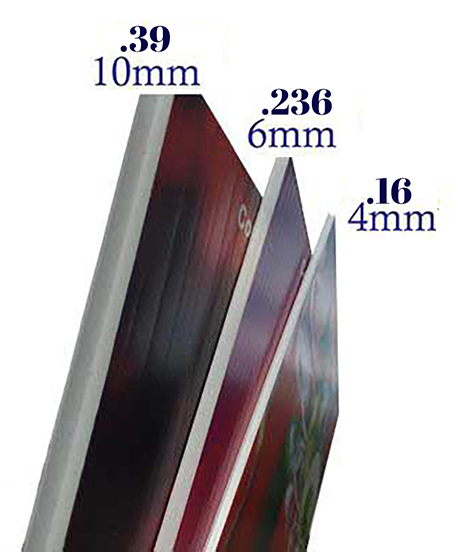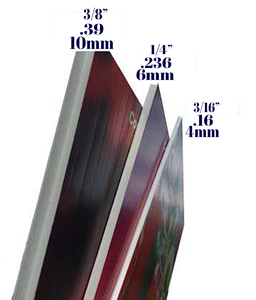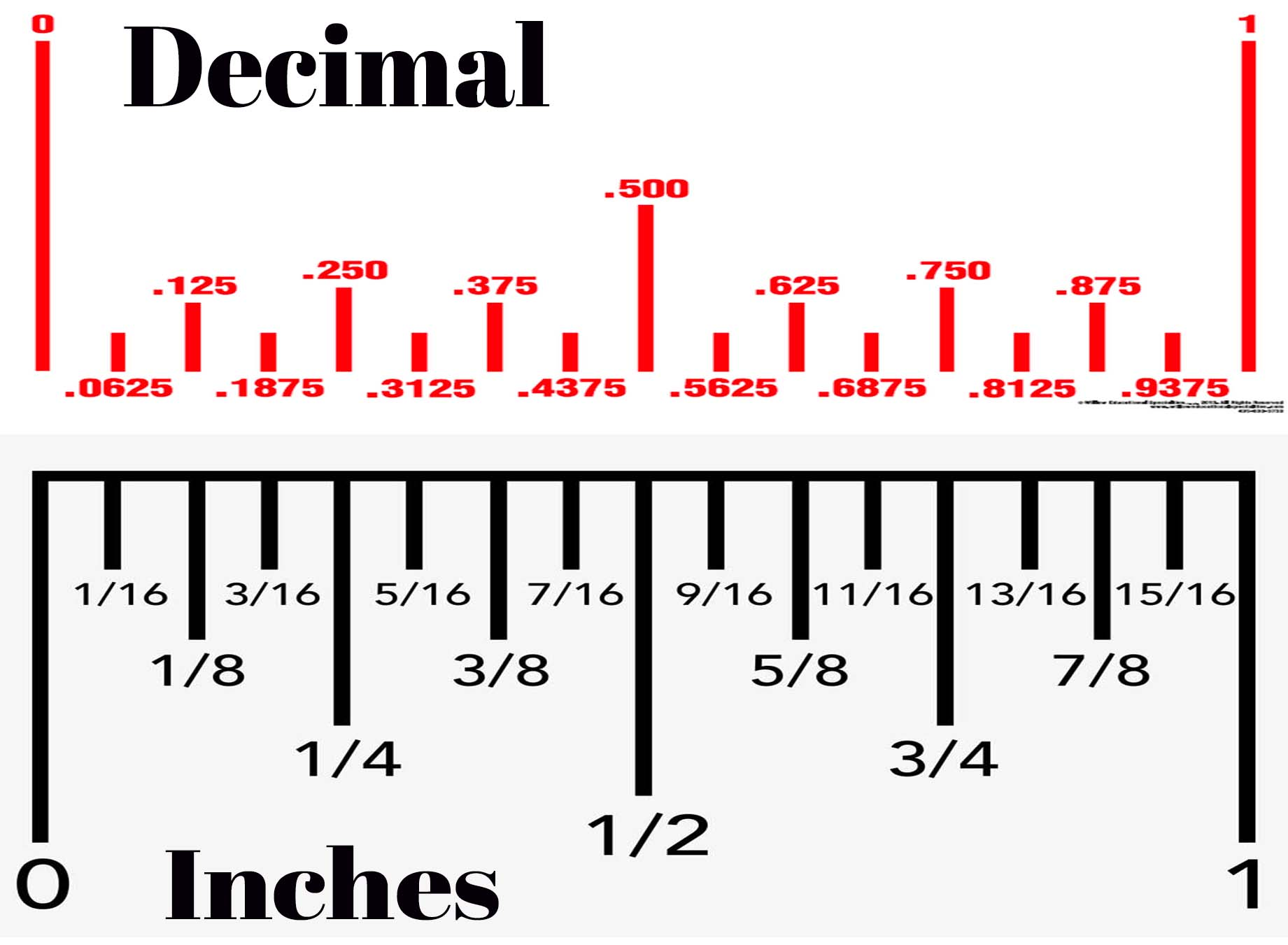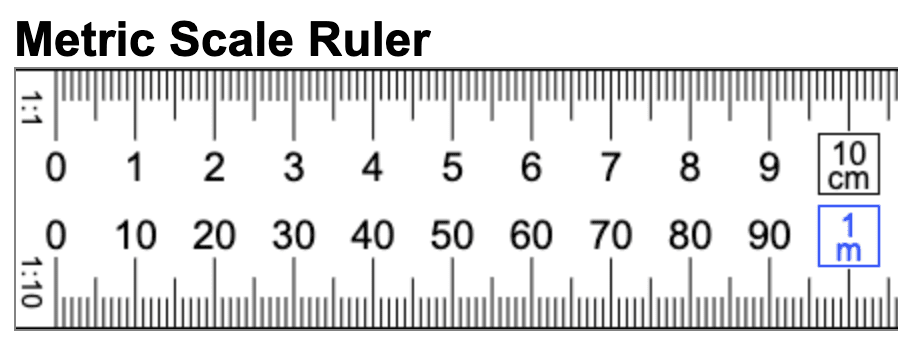How to convert inches to cm to mm to decimals to fractions

# How do I convert measurements? Inches to millimeters, millimeters to centimeters. And fractions to decimals.

One of the most common questions when ordering signs is how do I convert different measurements. Below are some images that should help to explain the difference. At the bottom of the page is a link to an amazing website where you can plug in your measurements and it will quickly convert it into every other measurement you need.

Converting measurements can be a challenging task, especially when dealing with different units of measurement such as centimeters, millimeters, inches, decimals, and fractions. However, with a basic understanding of each unit of measurement and the formulas used to convert them, the process can become much simpler.

Centimeters and millimeters are metric units of measurement commonly used in many countries around the world, while inches, decimals, and fractions are used primarily in the United States.

Centimeters and Millimeters: A centimeter is equal to 10 millimeters. To convert centimeters to millimeters, you can simply multiply the number of centimeters by 10. For example, if you have a measurement of 5 centimeters, the equivalent measurement in millimeters would be 50 millimeters (5 x 10 = 50).

Inches An inch is equal to 2.54 centimeters. To convert inches to centimeters, you can multiply the number of inches by 2.54. For example, if you have a measurement of 10 inches, the equivalent measurement in centimeters would be 25.4 centimeters (10 x 2.54 = 25.4).

Decimals: Decimals are a common way of expressing measurements in the United States. A decimal represents a part of a whole number, and it can be converted into inches or centimeters using the following formulas:

• To convert decimal inches to centimeters, you can multiply the number of decimal inches by 2.54. For example, if you have a measurement of 4.5 inches, the equivalent measurement in centimeters would be 11.43 centimeters (4.5 x 2.54 = 11.43).
• To convert decimal inches to fractions of an inch, you can multiply the decimal by 16 and round to the nearest fraction. For example, if you have a measurement of 3.25 inches, you can multiply 3.25 by 16 to get 52. The nearest fraction would be ½, so the measurement would be 3 ½ inches.

Fractions: Fractions are another common way of expressing measurements in the United States. Fractions can be converted into decimal inches or centimeters using the following formulas:

• To convert fractions of an inch to decimal inches, you can divide the numerator (top number) by the denominator (bottom number). For example, if you have a measurement of 3/8 inch, you can divide 3 by 8 to get 0.375 inches.
• To convert fractions of an inch to centimeters, you can convert the fraction to decimal inches and then use the formula for converting decimal inches to centimeters. For example, if you have a measurement of 1 ¼ inches, you can convert it to a decimal by adding 1 to ¼ (which is 0.25) to get 1.25 inches. You can then multiply 1.25 by 2.54 to get the equivalent measurement in centimeters, which is 3.175 centimeters.

Converting measurements requires an understanding of the different units of measurement and the formulas used to convert them. With practice, converting measurements can become easier and more intuitive, allowing you to work with different units of measurement confidently and accurately.

Centimeters: Centimeters are a unit of measurement in the metric system. They are used to measure length or distance and are abbreviated as "cm". One centimeter is equal to 0.01 meters or 10 millimeters.

Millimeters: Millimeters are also a unit of measurement in the metric system. They are used to measure length or distance and are abbreviated as "mm". One millimeter is equal to 0.1 centimeters or 0.001 meters.

Inches: Inches are a unit of measurement in the imperial system, commonly used in the United States. They are used to measure length or distance and are abbreviated as "in" or ". One inch is equal to 2.54 centimeters.

Decimals: Decimals are a way of expressing fractions as a decimal number. They are commonly used in the United States to express measurements in inches. For example, 1/2 inch can be expressed as 0.5 inches.

Fractions: Fractions are another way of expressing measurements in the United States. They are often used to express measurements in inches, where the numerator represents the number of parts and the denominator represents the total number of parts in the whole. For example, 3/4 inch means three out of four parts of an inch.

What size sign should I get? Many of our sign products are measured in milimeters (mm), decimals and inches. If you're not familiar with milimeters you may not know how thick your sign will be. Hopefully the information on this page will help you interpret and convert the different measurements!Below is a comparison of Decimals and Inches. The images are lined up with each other so you can see that 1/4" lines up with .250 in decimals. And .500 decimals lines up with 1/2".Next, the image below shows a conversion between Inches, Milimeters and Centimeters:
And for those who use the Metric measuring system:If you just need to find a quick measurement FAST, click on the photo below!

#### TAGS

convert measurementsmeasurementsruler